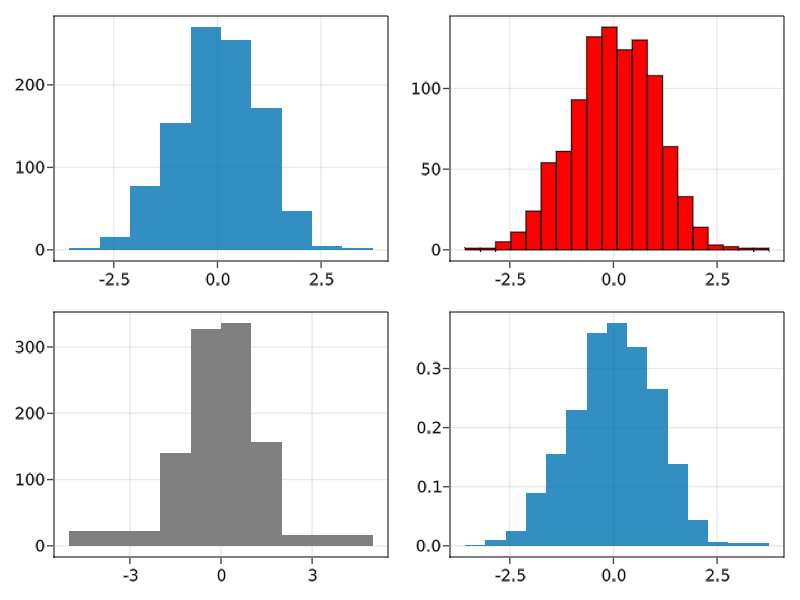# hist

Makie.histFunction
hist(values; bins = 15, normalization = :none)

Plot a histogram of values. bins can be an Int to create that number of equal-width bins over the range of values. Alternatively, it can be a sorted iterable of bin edges. The histogram can be normalized by setting normalization. Possible values are:

• :pdf: Normalize by sum of weights and bin sizes. Resulting histogram has norm 1 and represents a PDF.
• :density: Normalize by bin sizes only. Resulting histogram represents count density of input and does not have norm 1. Will not modify the histogram if it already represents a density (h.isdensity == 1).
• :probability: Normalize by sum of weights only. Resulting histogram represents the fraction of probability mass for each bin and does not have norm 1.
• :none: Do not normalize.

Attributes

Available attributes and their defaults for Combined{Makie.hist, T} where T are:

  bins           15
cycle          [:color => :patchcolor]
normalization  :none
source

### Examples

using GLMakie

data = randn(1000)

f = Figure()
hist(f[1, 1], data, bins = 10)
hist(f[1, 2], data, bins = 20, color = :red, strokewidth = 1, strokecolor = :black)
hist(f[2, 1], data, bins = [-5, -2, -1, 0, 1, 2, 5], color = :gray)
hist(f[2, 2], data, normalization = :pdf)
f